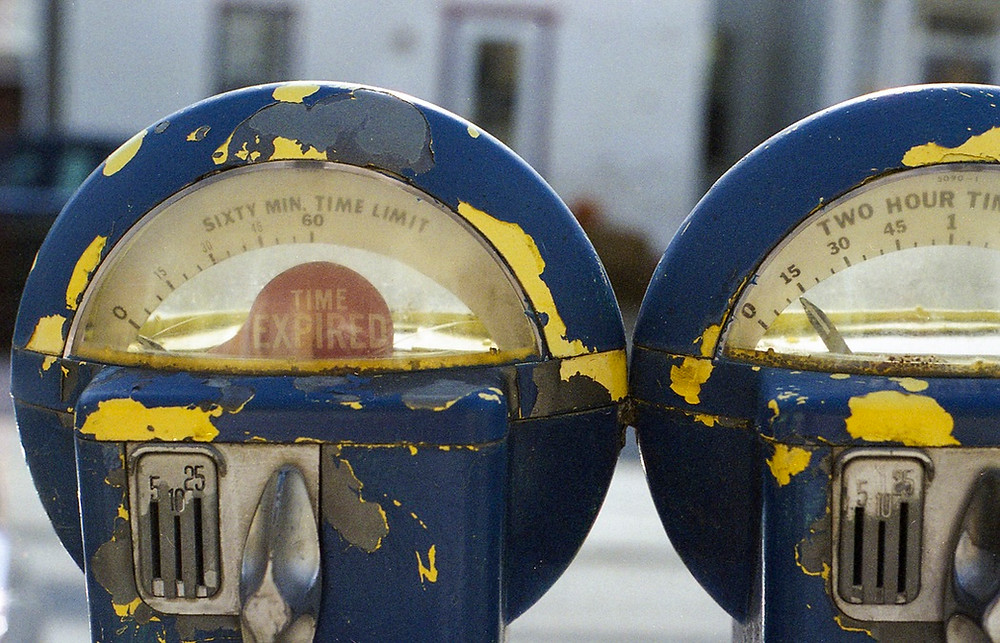# Options Trading VI, Gamma Distribution in relation to TimeThis publication is part of a series of posts on the Greeks. These posts are a short summary of a part of the content of my book How to Calculate Options Prices and Their Greeks.

In Options Trading V, Gamma Distribution in relation to Volatility, we’ve shown how the Gamma changes at different Volatility levels. As a rule of thumb we can say:

For at the money options: the higher the Volatility, the lower the Gamma

For out of the money options: the higher the Volatility, the higher the Gamma

The chart belows shows these features:For the Gamma Distribution in relation to Time to Maturity we can philosophise on the back of the Cone, as I have used in several articles for showing the impact of Time and Volatility on the evolution of the price of an asset.For constructing the cone, one standard deviation will be represented by:The upper boundary will be 4 standard deviations away from the at the money level, representing 99,999% of the probability distribution. The lower boundary will have the same ratio as the upper boundary compared to the at the money level (due to the lognormal distribution). In this case, as shown in the chart above, at 1 year Time to Maturity, the upper level is at 70, being four times 10% times the square root of 1 times 50 away from the \$50 starting level; as a result of the lognormal convention, the lower boundary will be set at 50 divided by 1.4 (the ratio of 70 compared to 50) resulting in a level of around \$35.7

Knowing that optionality is only there when being within the boundaries of the Cone, we can conclude that with one year to Maturity and at a Volatility of 10%, the 0 to 100% delta change will be in the range between 35.7 and 70. Outside these boundaries the Delta doesn’t change anymore and thus Gamma will be zero. As a result, within the boundaries there will be Gamma and its surface area must represent 100% (the Delta has a maximum change of 100%).

Comparing this with a Time to Maturity of a quarter of a year, this range will be between 60 (four times 10% times square root of a quarter times 50 away from the \$50 level) and 41.67 (50 divided by 1.2), as shown below.So when comparing the Gamma for 1 Year to Maturity with the Gamma for a Quarter of a Year, one can say that for 1 Year to Maturity the Gamma is distributed over a much larger range (around \$34) as compared to the latter (around \$18). Having the surface area for both at 100% must imply that for longer term options the Gamma around the at the money options should be lower than the Gamma for options with a shorter Time to Maturity, in order to keep the surface area constant at 100%.

Below is shown how the Gamma is distributed for these two different times to maturity:So as a conclusion, we can come up with the following rules of thumb:

For at the money options: the longer the Time to Maturity, the lower the Gamma

For out of the money options: the longer the Time to Maturity, the higher the Gamma

###### Search By Tags
Er zijn nog geen tags.